# Real number calculator

## Result:

### -5 = -5

The calculator makes basic and advanced operations with real numbers, natural numbers, integers, rational and irrational numbers. It also shows detailed step-by-step information about calculation procedure. Solve problems with two, three, or more real numbers in one expression. Add, subtract, and multiply real numbers step-by-step. This calculator performs addition, subtraction, multiplication, or division for calculations on positive or negative real numbers. This online real number calculator will help you understand how to add, subtract, multiply, or divide real numbers.

Real numbers are numbers that can be found on the number line. This includes the natural numbers( 1,2,3 ...), integers (-3) rational (fractions) and irrational numbers (like √2 or π). Positive or negative, large or small, whole numbers or decimal numbers are all real numbers. Imaginary numbers and complex numbers cannot be draw in number line, but in complex plane.

#### Examples:

addition of real numbers: -3/2 + pi/2
subtraction of real numbers: 32.14 - 67.32
parenthesis: sin(pi/2) * (1.1+2.2+3.3)
Complex example: Priority of operators and brackets: 3⋅[8−(−1) + (−3)÷(−3)]^2

## Real numbers in word problems:

• Reciprocal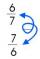Forgiven real numbers, calculate the reciprocal numbers.
• Exponential equationSolve exponential equation (in real numbers): 98x-2=9
• Mixing operations with numbersSubtract twice the number -23.6 from the difference of the numbers -130 and -40.2.Determine the numbers b, c that the numbers x1 = -1 and x2 = 3 were roots of quadratic equation: ?
• Imaginary numbersFind two imaginary numbers whose sum is a real number. How are the two imaginary numbers related? What is its sum?
• Distance of numbersWhich number has the same distance from the numbers -5.65 and 7.25 on the numerical axis?
• Is equal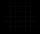Is equal following terms? ?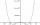By introducing a new variable solve biquadratic equation: ?
• Logarithmic equation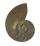Solve equation: log33(3x + 21) = 0
• Factors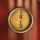Can the expression ? be factored into rational factors?
• Real estate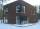Residential house has three entrances numbered by odd numbers arithmetic progression. The sum of the two numbers on the corner entrances is 50. Calculate the highest of this three numbers.
• Root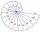Use law of square roots roots: ?
• CotangentIf the angle α is acute, and cotg α = 1/3. Determine the value of sin α, cos α, tg α.
• Cube root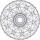Find cube root of 18
• Coordinate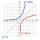Determine missing coordinate of the point M [x, 120] of the graph of the function f bv rule: y = 5x
• Geometric mean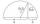Calculate the geometric mean of numbers a=15.2 and b=25.6. Determine the mean by construction where a and b are the length of the lines.
• Events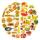Event P has probability of 0.84. What is the probability that the event P occurs in 3, 5, 7 try.